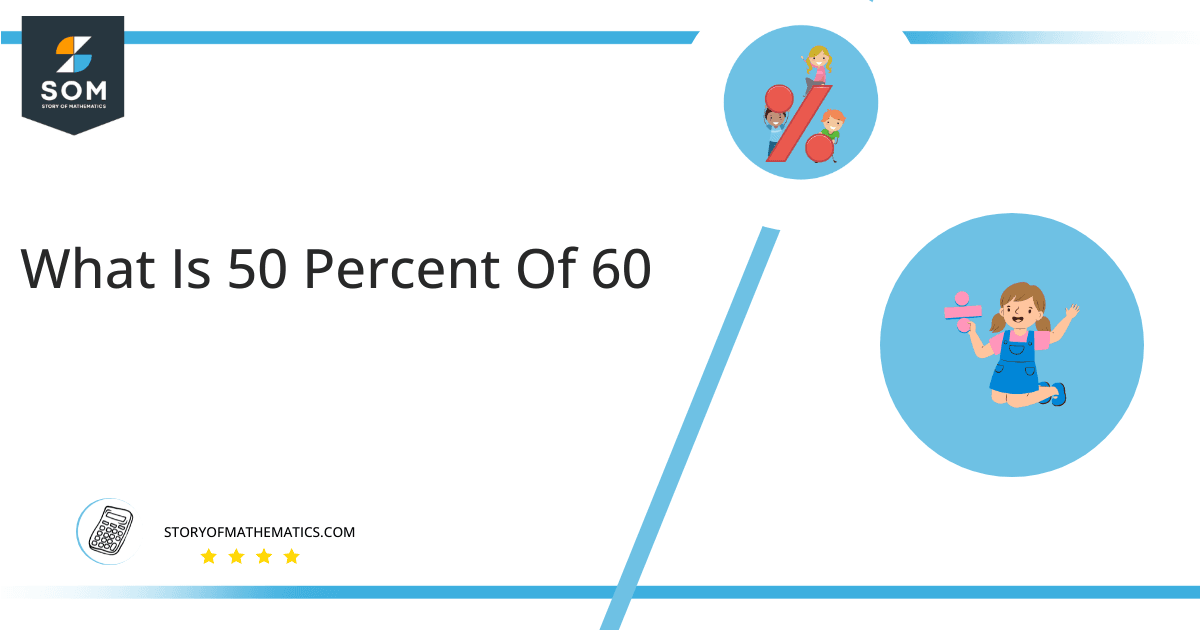# What Is 50 Percent of 60 + Solution with Free Steps?The 50 percent of 60 is equal to 30. It can be easily calculated by dividing 50 by 100 and multiplying the answer with 60 to get 30.

The easiest way to get this answer is by solving a simple mathematical problem of percentage. You need to find 50% of 60 for some sale or real-life problem. Divide 50 by 100, multiply the answer with 60, and get the 50% of 60 value in seconds.

This article will explain the full process of finding any percentage value from any given quantity or number with easy and simple steps.

## What Is 50 percent of 60?

The 50 percent of 60 is 30.

The percentage can be understood with a simple explanation. Take 60, and divide it into 100 equal parts. The 50 number of parts from the total 100 parts is called 50 percent, which is 30 in this example.

## How To Calculate 50 percent of 60?

You can find 50 percent of 60 by some simple mathematical steps explained below.### Step 1

Firstly, depict 50 percent of 60 as a fractional multiple as shown below:

50% x 60

### Step 2

The percentage sign % means percent, equivalent to the fraction of 1/100.

Substituting this value in the above formula:

= (50/100) x 60

### Step 3

Using the algebraic simplification process, we can arithmetically manipulate the above equation as follows:

= (50 x 60) / 100

= 3000 / 100

= 30This percentage can be represented on a pie chart for visualization. Let us suppose that the whole pie chart represents the 60 value. Now, we find 50 percent of 60, which is 30. The area occupied by the 30 value will represent the 50 percent of the total 60 value. The remaining region of the pie chart will represent 50 percent of the total 60 value. The 100% of 60 will cover the whole pie chart as 60 is the total value.

Any given number or quantity can be represented in percentages to better understand the total quantity. The percentage can be considered a quantity that divides any number into hundred equal parts for better representation of large numbers and understanding.

Percentage scaling or normalization is a very simple and convenient method of representing numbers in relative terms. Such notations find wide application in many industrial sectors where the relative proportions are used.